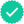Question

1. Estimate the volume of the solid that lies below the surface z = xy and above the following rectangle. R = (x, y) | 6 ≤ x ≤ 12, 8 ≤ y ≤ 12

(a) Use a Riemann sum with m = 3, n = 2, and take the sample point to be the upper right corner of each square.

(b) Use the Midpoint Rule to estimate the volume of the solid.

2.Calculate the double integral. 10(1 + x^2) / (1 + y^2) dA, R = {(x, y) | 0 ≤ x ≤ 3, 0 ≤ y ≤ 1} R

Expert SolutionStudents who’ve seen this question also like:Your question is solved by a Subject Matter ExpertWant to see this answer and more?
Experts are waiting 24/7 to provide step-by-step solutions in as fast as 30 minutes!*
*Response times may vary by subject and question complexity. Median response time is 34 minutes for paid subscribers and may be longer for promotional offers.8+ million solutions